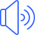number

المضارع او المصدرnumbered

الماضيnumbered

التصريف الثالثnumbering

صيغة الـ ing

# استخدامات الفعل number مع جميع الازمنة الانجليزية

Simple Present Tense

# زمن المضارع البسيط

I number
You number
He numbers
She numbers
It numbers
We number
You number
They number

لا يفوتك تحميل تطبيقنا المجاني الشامل والذي سوف يغنيك عن كل شي حيث سوف يبدء معك من مستوى الاحرف الانجليزية ويستمر معك الى مستوى الاحتراف بأذن الله مع اختبارات تفاعلية متعددة وشرح مفصل لكل صغيرة وكبيرة علما ان جميع الدروس والمقاطع الصوتية تعمل بدون الحاجة الى الانترنت. ولاتنسى تقيم التطبيق اذا كنت بالفعل حملته على جهازك

تطبيق الايفون تطبيق الاندرويد

Simple Past Tense

# زمن الماضي البسيط

I numbered
You numbered
He numbered
She numbered
It numbered
We numbered
You numbered
They numbered

Simple Future Tense

# زمن المستقبل البسيط

I will number
You will number
He will number
She will number
It will number
We will number
You will number
They will number

Present Perfect Tense

# زمن المضارع التام

I have numbered
You have numbered
He has numbered
She has numbered
It has numbered
We have numbered
You have numbered
They have numbered

Past Perfect Tense

# زمن الماضي التام

Future Perfect Tense

# زمن المستقبل التام

I will have numbered
You will have numbered
He will have numbered
She will have numbered
It will have numbered
We will have numbered
You will have numbered
They will have numbered

Present Continuous Tense

# زمن المضارع المستمر

I am numbering
You are numbering
He is numbering
She is numbering
It is numbering
We are numbering
You are numbering
They are numbering

Past Continuous Tense

# زمن الماضي المستمر

I was numbering
You were numbering
He was numbering
She was numbering
It was numbering
We were numbering
You were numbering
They were numbering

Future Continuous Tense

# زمن المستقبل المستمر

I will be numbering
You will be numbering
He will be numbering
She will be numbering
It will be numbering
We will be numbering
You will be numbering
They will be numbering

Present Perfect Continuous Tense

# زمن المضارع التام المستمر

I have been numbering
You have been numbering
He has been numbering
She has been numbering
It has been numbering
We have been numbering
You have been numbering
They have been numbering

Past Perfect Continuous Tense

# زمن الماضي التام المستمر

Future Perfect Continuous Tense

# زمن المستقبل التام المستمر

I will have been numbering
You will have been numbering
He will have been numbering
She will have been numbering
It will have been numbering
We will have been numbering
You will have been numbering
They will have been numbering

لكي تتابع دروسنا الجديدة بالصوت والصورة والشرح المفصل انصحك بالاشتراك في قناتنا على اليوتيوب

او انشر الصفحة على مواقع التواصل الاجتماعي

### كلمات دالة

number تصريف فعل

numbered تصريف الفعل

numbered تصريف فعل

number تصريف الفعل

number ترجمة و معنى كلمة

number معنى الفعل

number ترجمة الفعل